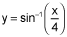# 5 Short Answer Calc Questions 1

### Question Description

1.

Find the area of the region bounded by the curves, y = 0, and x = 4 obtained by integrating with respect to y. Your work must include the definite integral and the antiderivative. (10 points)

2.

Set up, but do not evaluate, the integral which gives the volume when the region bounded by the curves y = Ln(x), y = 1, and x = 1 is revolved around the line y = -3. (10 points)

3.

The base of a solid in the xy-plane is the first-quadrant region bounded y = x and y = x2. Cross sections of the solid perpendicular to the x-axis are semicircles. What is the volume, in cubic units, of the solid? (10 points)

4.

Determine if the Mean Value Theorem for Integrals applies to the function f(x) = x3 – 4x on the interval [-1, 1]. If so, find the x-coordinates of the point(s) guaranteed to exist by the theorem. (10 points)

5.

An object has a constant acceleration of 40 ft/sec2, an initial velocity of -20 ft/sec, and an initial position of 10 ft. Find the position function, s(t), describing the motion of the object. (10 points

1.Find the area of the region bounded by the curves , y = 0, and x = 4 obtained by integrating with respect to y. Your work must include the definite integral and the antiderivative. (10 points)2.Set up, but do not evaluate, the integral which gives the volume when the region bounded by the curves y = Ln(x), y = 1, and x = 1 is revolved around the line y = -3. (10 points)3.The base of a solid in the xy-plane is the first-quadrant region bounded y = x and y = x2. Cross sections of the solid perpendicular to the x-axis are semicircles. What is the volume, in cubic units, of the solid? (10 points)4.Determine if the Mean Value Theorem for Integrals applies to the function f(x) = x3 – 4x on the interval [-1, 1]. If so, find the x-coordinates of the point(s) guaranteed to exist by the theorem. (10 points)5.An object has a constant acceleration of 40 ft/sec2, an initial velocity of -20 ft/sec, and an initial position of 10 ft. Find the position function, s(t), describing the motion of the object. (10 points